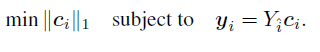# Convex problems

Hi,all.When I am using the cvx tools to solve the problemsomething confusing happended.i.e,
for every observation value yi,the optimal vector xi is a zero vector.
Is there anyone who could tell me what’s wrong?Following is my codes for finding the optimal vector for y3,and C is a given 100x200 matrix.

``````n=size(C,1);
p=size(C,2);
A=eye(size(C,2));
d=C(:,3);
C(:,3)=[];
cvx_begin
cvx_precision best
variable x(p-1)
minimize( norm(x,1) )
subject to
d=C*x
cvx_end
~~~~~~~~~~~~~~~~~~~~~~~~
I'd appreciate it if you guys could help me out.Thanks again!``````

I think you want

``````d==C*x
``````

not

``````d=C*x
``````

You need == for an equality constraint. Using a single =, your d is a CVX expression, C*x, and has nothing to do with your d which was C(:,3).

Because your problem as incorrectly entered is unintentionally unconstrained, the zero vector is always optimal.

1 Like

Mark,
Thank you a lot.I have solved the problem above.
However,I am Now having big troubles,stuck in MOTION SEGMENTATION ALGORITHMS.
More specifically,motion segmentation refers to the problem of separating a video sequence into multiple spatiotemporal regions corresponding to different rigid-body motions.
Coding is some kind of art.That’s what I got these days.